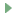# Scientific mode tutorial

In this tutorial, you operate in Scientific Mode and use Matplotlib and NumPy packages to run and debug a Python code with data visualization.

Before you start, ensure the following is installed:

## Creating a Scientific project

### Create a PyCharm project with the scientific settings predefined

1. Open the New Project wizard (File | New | Project).

2. Choose Scientific project type.

3. In the project settings dialog window, specify a project name, ensure the Conda is selected as the new environment, and alter the default data folder (if needed), then click Create.

4. Open the main.py file and add the following code sample:

import numpy as np import matplotlib.pyplot as plt N = 50 x = np.random.rand(N) y = np.random.rand(N) colors = np.random.rand(N) area = np.pi * (15 * np.random.rand(N))**2 # 0 to 15 point radii plt.scatter(x, y, s=area, c=colors, alpha=0.5) plt.show() X = np.linspace(-np.pi, np.pi, 256,endpoint=True) C,S = np.cos(X), np.sin(X) plt.plot(X, C, color="blue", linewidth=2.5, linestyle="-") plt.plot(X, S, color="red", linewidth=2.5, linestyle="-") plt.xlim(X.min()*1.1, X.max()*1.1) plt.xticks([-np.pi, -np.pi/2, 0, np.pi/2, np.pi], [r'$-\pi$', r'$-\pi/2$', r'$0$', r'$+\pi/2$', r'$+\pi$']) plt.ylim(C.min()*1.1,C.max()*1.1) plt.yticks([-1, 0, +1], [r'$-1$', r'$0$', r'$+1$']) plt.show()
5. Process warnings shown for the numpy and matplotlib imports and enable the packages in the project.

## Running

Run your scientific project Shift+F10. The code is executed and shows two graphs in the SciView. Clicking the preview thumbnail displays the respective graph:

You can modify the project code to plot only one graph at a time. In the scientific mode, you can execute fragments of your code by creating code cells.

### To execute code cells:

1. Modify the main.py file by adding the "#%%" lines.

2. In the gutter, click the iconCtrl+Enter on line with the scatter plot cell mark. Only the scatter graph will be built.

3. Now click the iconor press Ctrl+Enter on the line with the y versus x plot cell mark. The corresponding graph should appear.

## Debugging

Let's put a breakpoint at the line:

plt.show()

This line appears twice in our example code, and so there will be two breakpoints.

Right-click the editor background and from the context menu choose Debug 'main'.

You see the Debug tool window and the grey characters in the editor. This is the result of the inline debugging, which is enabled.

The line with the first breakpoint is blue highlighted. It means that the debugger has stopped at the line with the breakpoint, but has not yet executed it. If we execute this line (for example, by clicking thebutton on the stepping toolbar of the Debug tool window), we'll see the graph:

Next, look at the Variables tab of the Debug tool window. If you click the View as Array link nearby the area array, the Data tab in the SciView window opens:

When you process excessive amount of data, you might experience degradation of debugging performance if the debugger loads variable's values synchronously or asynchronously. It is recommended to switch to the On demand mode by selecting the corresponding loading policy. See Managing Variables Loading Policy for more information.

Mind the only row of figures in the Data tab in the SciView - it's explained by the fact that the area array is one-dimensional.

## Running in console

Right-click the editor background and choose the Run File in Console command:

This command corresponds to running a run/debug configuration for the main.py file with the Run with Python console checkbox selected:

When this command is run, the >>> prompt appears after the output in the Run tool window, and you can execute your own commands.

## Summary

So, what has been done with the help of PyCharm?

• The file main.py was created and opened for editing.

• The source code has been entered (note the powerful PyCharm's code completion!)

• The source code has been run and debugged.

• Finally, we ran the file in console.